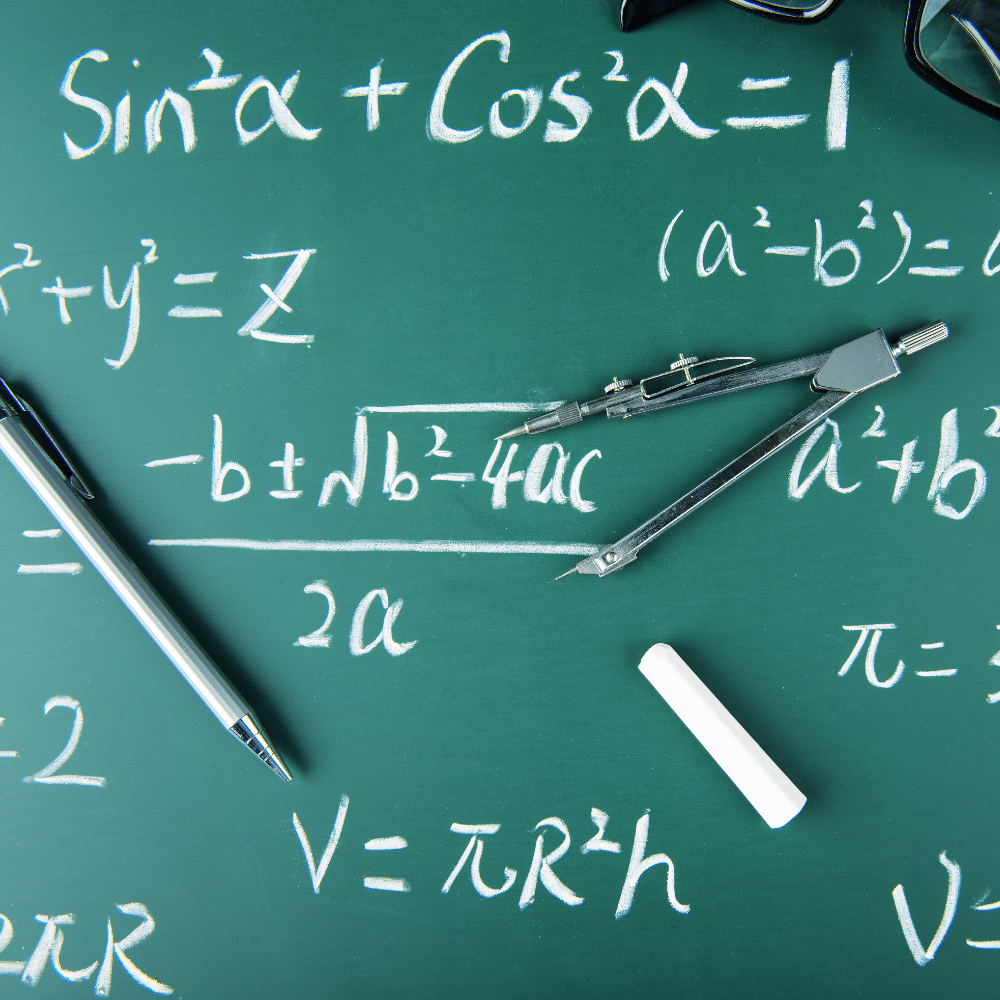# Maths Tuition

Get ahead of the pack.

Tackle any Maths question you have and gain the skills to ace the exams
with TigerCampus Hong Kong’s private tuition service.## Overview

### Customised curriculum

Choose one or more subjects, and we'll find a tutor who can make sure you're prepared.

### Flexible

Take lessons only when you need them—as little or as many as necessary until you feel confident.

### Private lesson

No need to accommodate other students. Learning is customised your perfect pace and difficulty so you are always improving.

## Maths Education in Hong Kong

Mathematics competency is the important basis for technical and science education at the higher levels, according to the Hong Kong educational system.

Elementary mathematics is a required topic at the Standard and Form levels, and maths tutoring professionals in Hong Kong may help students develop an interest in the subject from an early age.

## Description

TigerCampus Hong Kong is one of the premium online tuition centers in the country. We have at-home and online Maths tutors. TigerCampus tutors are able to provide quality home and online tuition to prepare you to take any maths exam. From primary level up until university, we provide tuition for any maths tuition you find necessary.

Check out our available syllabuses below and request a free trial today!

## Benefits

### Our available maths tuition syllabuses

##### Year 1
1. Number, algebra, statistics and geometry
2. Number, algebra and graphs
3. Mensuration, probability and statistics
4. Trigonometry, geometry and statistics
5. Algebra and graphs
6. Geometry and vectors
##### Year 2
1. Number, algebra, graphs and geometry
2. Number, probability, algebra, geometry and trigonometry
3. Algebra and graphs
4. Trigonometry, vectors, geometry, statistics, algebra and graph
##### Year 1
1. Functions
2. Quadratic equations and inequalities
3. Indices, surds and polynomials
4. Simultaneous equations
5. Logarithms and exponential functions
6. Straight line graphs and vectors
7. Permutations, combinations and series
##### Year 2
1. Permutations, combinations and series (continued)
2. Trigonometry
3. Differentiation
4. Integration
##### Form 4
1. Number systems
2. Equations of straight lines
3. Quadratic equations in one unknown
4. Functions and graphs
5. Exponential and logarithmic functions
6. Rational functions
7. Properties of circles
8. Polynomials
9. Trigonometry
##### Form 5
1. Variations
2. Equations
3. Inequalities
4. Graphs of functions
5. Permutation and combination
6. Probability
7. Equations of circles and locus
8. Solving triangles
9. Applications in trigonometry
10. Measures of dispersion

##### Form 6
1. Arithmetic and geometric sequences
2. Linear inequalities in two unknowns
3. Linear programming
4. Uses and abuses of statistics
##### Pure Mathematics 1 (Paper 1)
2. Functions
3. Coordinate geometry
4. Circular measure
5. Trigonometry
6. Series
7. Differentiation
8. Integration

##### Pure Mathematics 2 (Paper 2)
1. Algebra
2. Logarithmic and exponential functions
3. Trigonometry
4. Differentiation
5. Integration
6. Numerical solution of equations

##### Pure Mathematics 3 (Paper 3)
1. Algebra
2. Logarithmic and exponential functions
3. Trigonometry
4. Differentiation
5. Integration
6. Numerical solution of equations
7. Vectors
8. Differential equations
9. Complex numbers

##### Mechanics
1. Forces & equilibrium
2. Kinematics of motion in a straight line
3. Momentum
4. Newton’s laws of motion
5. Energy, work and power

##### Probability & Statistics 1
1. Representation of data
2. Permutations and combinations
3. Probability
4. Discrete random variables
5. The normal distribution

##### Probability & Statistics 2
1. The Poisson distribution
2. Linear combinations of random variables
3. Continuous random variables
4. Sampling and estimation
5. Hypothesis tests
##### Further Pure Mathematics 1
1. Roots of polynomial equations
2. Rational functions and graphs
3. Summation of series
4. Matrices
5. Polar coordinates
6. Vectors
7. Proof by induction

##### Further Pure Mathematics 2
1. Hyperbolic functions
2. Matrices
3. Differentiation
4. Integration
5. Complex numbers
6. Differential equations

##### Further Mechanics
1. Motion of a projectile
2. Equilibrium of a rigid body
3. Circular motion
4. Hooke’s law
5. Linear motion under a variable force
6. Momentum

##### Further Probability & Statistics
1. Continuous random variables
2. Inference using normal and t-distributions
3. χ² tests
4. Non-parametric tests
5. Probability generating functions
##### Analysis and Approaches (SL)
1. Number & Algebra
2. Functions
3. Geometry & Trigonometry
4. Probability & Statistics
5. Calculus
6. Toolkit & Exploration

##### Applications and Interpretation (SL)
1. Number & Algebra
2. Functions
3. Geometry & Trigonometry
4. Probability & Statistics
5. Calculus
6. Toolkit & Exploration
##### Analysis and Approaches (HL)
1. Number & Algebra
2. Functions
3. Geometry & Trigonometry
4. Probability & Statistics
5. Calculus
6. Toolkit & Exploration

##### Applications and Interpretation (HL)
1. Number & Algebra
2. Functions
3. Geometry & Trigonometry
4. Probability & Statistics
5. Calculus
6. Toolkit & Exploration
##### Our tutors graduated from top universitiesLeave your phone number, and we’ll call you back to discuss how we can help you.

## How it works

### 1Request a tutor

Let us know your goals and age range. We'll figure out a plan to help get you there.

### 2Match with a tutor

You'll get a tutor based on your needs and goals, or you can request a specific tutor.

### 3Start a free trial

Experience a free trial lesson with your new tutor and see if your learning style matches.

### 4Keep it up!

If everything went well, sign up to keep going! You can choose the pacing of the lessons

### 1Request a tutor

Let us know your goals and age range. We'll figure out a plan to help get you there.

### 2Match with a tutor

You'll get a tutor based on your needs and goals, or you can request a specific tutor.

### 3Start a free trial

Experience a free trial lesson with your new tutor and see if your learning style matches.

### 4Keep it up!

If everything went well, sign up to keep going! You can choose the pacing of the lessons